Courses

# Radiation - MCQ Test 1

## 20 Questions MCQ Test RRB JE for Mechanical Engineering | Radiation - MCQ Test 1

Description
This mock test of Radiation - MCQ Test 1 for Mechanical Engineering helps you for every Mechanical Engineering entrance exam. This contains 20 Multiple Choice Questions for Mechanical Engineering Radiation - MCQ Test 1 (mcq) to study with solutions a complete question bank. The solved questions answers in this Radiation - MCQ Test 1 quiz give you a good mix of easy questions and tough questions. Mechanical Engineering students definitely take this Radiation - MCQ Test 1 exercise for a better result in the exam. You can find other Radiation - MCQ Test 1 extra questions, long questions & short questions for Mechanical Engineering on EduRev as well by searching above.
QUESTION: 1

Solution:

Ans. (b)

QUESTION: 2

Solution:
QUESTION: 3

### Fraction of radiative energy leaving one surface that strikes the othersurface is called

Solution:

Ans. (c)

QUESTION: 4

Which one of the following modes of heat transfer would take placepredominantly, from boiler furnace to water wall?

Solution:

Ans. (c) In boiler, the energy from flame is transmitted mainly by radiation to water wall and radiant super heater.

QUESTION: 5

When α is absorbtivity, ρ is reflectivity and τ is transmisivity, then for diathermanous body, which of the following relation is valid?

Solution:

Ans. (c)

QUESTION: 6

Consider the diagram given above. Which one of the following is correct?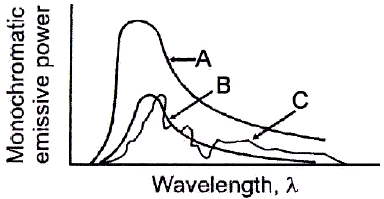Solution:

Ans. (d)

QUESTION: 7

Match List-I with List-II and select the correct answer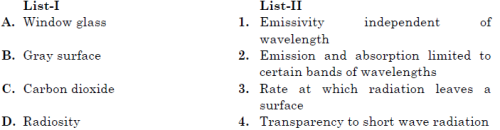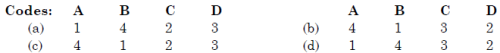Solution:

Ans. (c)

QUESTION: 8

Assertion (A): The nose of aeroplane is painted black. Reason (R) Black body absorbs maximum heat which is generated byaerodynamic heating when the plane is flying.

Solution:

Ans. (b)

QUESTION: 9

Match List-I with List-II and select the correct answer using the code given below the lists: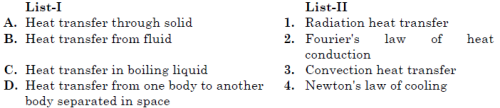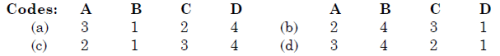Solution:

Ans. (b)
Heat transfer through solid → Fourier’s law of heat conduction
Heat transfer from hot surface to
surrounding fluid → Newton’s law of cooling
Heat transfer in boiling liquid → Convection heat transfer
Heat transfer from one body to → Radiation heat another transfer separated in space

QUESTION: 10

Two long parallel plates of same emissivity 0.75 are maintained at different temperatures and have radiation heat exchange between them. The radiation shield of emissivity 0.5 placed in the middle will reduce radiation heat exchange to:

Solution:

Ans. (c)

QUESTION: 11

Which of the following would lead to a reduction in thermal resistance?
1. In conduction; reduction in the thickness of the material and an increase in the thermal conductivity.
2. In convection, stirring of the fluid and cleaning the heating surface.
3. In radiation, increasing the temperature and reducing theemissivity.
Codes:

Solution:

Ans. (b) 1. In conduction, heat resistance = Δ x/kA
Thus reduction in thickness and increase in area result in reduction of thermal resistance.
2. Stirring of fluid and cleaning the heating surface increases value of h, and thus reduces thermal resistance.
3. In radiation, heat flow increases with increase in temperature and reduces with reduction in emissivity. Thus thermal resistance does not decrease.
Thus 1 and 2 are correct.

QUESTION: 12

Solar radiation of 1200 W/m2 falls perpendicularly on a grey opaquesurface of emissivity 0.5. If the surface temperature is 50°C and surfaceemissive power 600 W/m2, the radiosity of that surface will be:

Solution:

Ans. (c)

QUESTION: 13

For an opaque plane surface the irradiation, radiosity and emissive power are respectively 20, 12 and 10 W/m2.What is the emissivity of the surface?

Solution:

Ans. (c)
J = ε Eb + (1 − ε) G
12 = ε ×10 + (1 −ε ) × 20 or ε = 0.8

QUESTION: 14

Two large parallel grey plates with a small gap, exchange radiation atthe rate of 1000 W/m2 when their emissivities are 0.5 each. By coatingone plate, its emissivity is reduced to 0.25. Temperature remains unchanged. The new rate of heat exchange shall become:

Solution:

Ans. (b)

QUESTION: 15

Match List-I with List-II and select the correct answer using the code given below the Lists: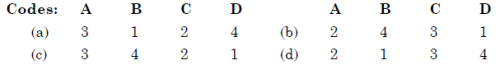Solution:

Ans. (b)

QUESTION: 16

The shape factor of a hemispherical body placed on a flat surface withrespect to itself is:

Solution:

Ans. (c)

QUESTION: 17

What is the value of the shape factor for two infinite parallel surface separated by a distance d?

Solution:

Ans. (c) All the emission from one plate will cross another plate. So Shape Factor in one.

QUESTION: 18

Intensity of radiation at a surface in perpendicular direction is equalto: [IES-2005; 2007]

Solution:

Ans. (c)
We know that,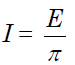QUESTION: 19

Sun's surface at 5800 K emits radiation at a wave-length of 0.5 μm. Afurnace at 300°C will emit through a small opening, radiation at awavelength of nearlyb

Solution:

Ans. (b)
As per Wien's law, λ1T12T2 or 5800 × 0.5 = λ2 × 57

QUESTION: 20

Match List-I with List-II and select the correct answer using the code given below the lists: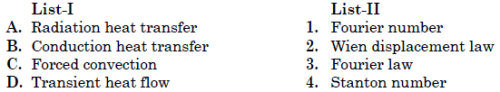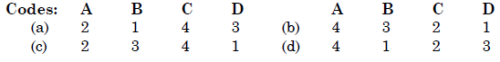Solution:

Ans. (c)